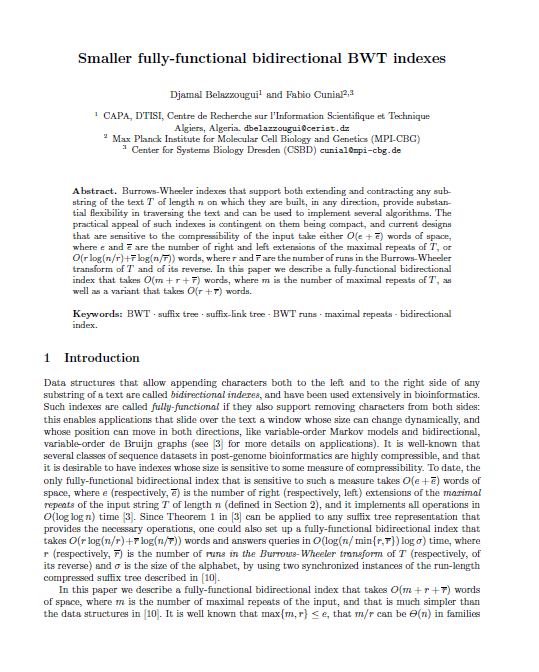## Smaller Fully-Functional Bidirectional BWT IndexesBurrows-Wheeler indexes that support both extending and contracting any substring of the text $T$ of length $n$ on which they are built, in any direction, provide substantial flexibility in traversing the text and can be used to implement several algorithms. The practical appeal of such indexes is contingent on them being compact, and current designs that are sensitive to the compressibility of the input take either $O(e+\REV{e})$ words of space, where $e$ and $\REV{e}$ are the number of right and left extensions of the maximal repeats of $T$, or $O(r\log(n/r)+\REV{r}\log(n/\REV{r}))$ words, where $r$ and $\REV{r}$ are the number of runs in the Burrows-Wheeler transform of $T$ and of its reverse. In this paper we describe a fully-functional bidirectional index that takes $O(m+r+\REV{r})$ words, where $m$ is the number of maximal repeats of $T$, as well as a variant that takes $O(r+\REV{r})$ words.